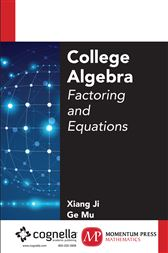# College Algebra

## Factoring and EquationsUS\$49.95
###### ISBNs
• 9781606508305
With a concise but rigorous approach, this group of four books on the fundamentals of algebra at the college level introduces the student to the full spectrum of mathematical tools that will serve him or her through all quantitative subjects at the university level, including as a preparation for first year calculus. Beginning with the basic elements of algebra including number theory and set theory, and going on through concepts of equations and functions and on to co-ordinate geometry and advanced algebraic expressions like polynomial equations, these books offer a comprehensive preparation for mathematics at the college level. Each of these four books presents clear explanations of fundamental concepts, followed by ample worked-out exercises to help reinforce the concepts as presented. This second book covers the basics of factoring, setting up linear equations, the uses for inequalities, and use of rational expressions and equations.

• Momentum Press; July 2015
• ISBN: 9781606508305